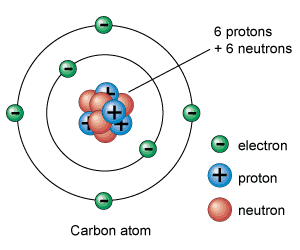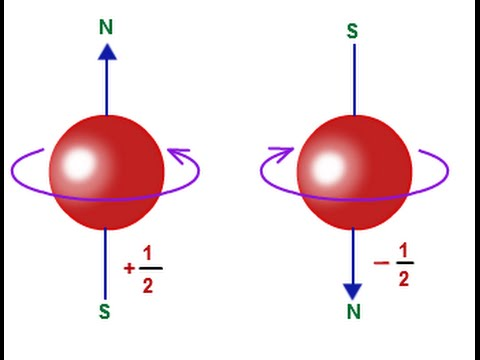# Electron Spin

## Electron Spin and Electron Spin Quantum Number

Introduction:

The position of each element in the periodic table gives important information about the structure of the elements, its properties, and behavior in the chemical reactions.

The periodic table helps you find out its electron configuration, to understand how the electrons are organized around the nucleus. The atoms use their electrons to take part in chemical reactions, so knowing an element’s electron configuration allows you to predict how it reacts whether, and how, it will interact with atoms of other elements.

Atom:Atoms are the fundamental units of matter and they define the structure of elements. The word indivisible is originated from a Greek word because it was once thought that atoms were the smallest things in the universe and these atoms cannot be divided further. We now know that atoms are made up of 3 particles: protons, neutrons, and electrons and these three particles are composed of even smaller particles such as quarks.

Nucleus:

The Ernest Rutherford discovered nucleus in the year 1911, a physicist from New Zealand, who in 1920 gave the name proton for the positively charged particles of the atom. Rutherford also said that there was also a neutral particle within the nucleus, which James Chadwick, a British physicist, was able to prove in 1932.

Virtually all the mass of the atom stays in the nucleus. The protons and neutrons that form up the nucleus are approximately the same mass such that the proton is slightly less and have the equal angular momentum, according to Lawrence Berkeley National Laboratory.

Protons:

Protons are positively charged particles seen within atomic nuclei. Rutherford saw them in experiments with cathode-ray tubes experiment between 1911 and 1919. Protons are somewhat smaller in mass than neutrons with a relative mass of 0.9986 such that as compared with the mass of the neutron being 1 or about 1.673x10-27 kg.

The total number of protons in an atom determines what element it is. For instance, carbon atoms have 6 protons, hydrogen atoms have 1 and oxygen atoms have 8. The number of protons in an atom is also referred to as the atomic number of that element. The total number of protons in an atom also defines the chemical behavior of the element. The Table of the Elements arranges elements in order of increasing atomic number.

Electrons:

Electrons are small compared to protons and neutrons, over 1,800 times less than either a proton or a neutron. Electrons have a relative mass of 0.0005439 such that the electron is compared with the mass of a neutron being one or about 9.109x10-31 kg.
The electron was discovered by a British physicist, J.J. Thomason, in the year 1897. Basically known as "corpuscles," electrons have a negative (-ve) charge and are electrically pulled to the positively charged protons. The atomic nucleus is surrounded by electrons in pathways called orbitals, this idea was put forth by Erwin Schrödinger, a physicist, in the 1920s. Now, this model is known as the quantum model or the electron cloud system. The inner orbitals surrounding the atom are Spherical, but the outer orbitals are much more complex.

An atom's electron configuration is the orbital description of the positions of the electrons in a typical atom. Using the electron configuration and laws of physics, chemists can predict an atom's properties, such as stability, boiling point, and conductivity.

Neutrons:

The neutron is used as a comparison to detect the relative mass of protons and electrons such that, so it has a relative mass of 1 and has a physical mass of 1.6749x10-27 kg.

The neutron's presence was theorized by Rutherford in 1920 and found by Chadwick in 1932. Neutrons were found during experiments when atoms were shot at a thin layer of beryllium. Subatomic particles with no charge have released the neutron.

After understanding what atom, nucleus, protons, neutrons, and neutrons is. Now let us discuss electron spin.

Electron Spin:

Electron spin is a quantum feature of electrons. It is a kind of angular momentum. The magnitude value of this angular momentum is permanent. Like charge and rest mass, spin is a basic, unvarying property of the electron.
As a teaching method, we can sometimes liken electron spin to the earth spinning on its own axis every 24 hours. If the electron spins clockwise on its axis, it is called as spin-up and it is counterclockwise then it is called spin-down. This is a suitable explanation, if not fully justifiable mathematically.

The spin angular momentum linked with electron spin is independent of orbital angular momentum, which is associated with the electrons that travel around the nucleus.

Electron spin is not used to determine the electron shells, subshells, or orbitals, unlike the quantum numbers n, l, and ml.

Electron spin is given by the equation, ∥S∥=√s * (s+1) * h.

Where,
s is the quantized spin vector
The spin vector is denoted by ||s||
The spin quantum number s is relative with the spin angular momentum
h is the Planck’s constant, h = 6.62607004 × 10-34 m2 kg / sIn the quantum theory, the electron is considered like the minute magnetic bar and its spin faces the north pole of the minute bar. If two proximate electrons have an alike spin direction, the magnetic field that is formed by them strengthens each other and hence a strong magnetic field is gained. If the proximate electrons have an opposite spin direction, and the magnetic field created by them cancels each other and no magnetic field is existent.

The spin quantum number is S = n / 2

n is said to be a non-negative integer.
The values of the spins are 0, 1/2, 1, 3/2, 2, etc.
The total angular momentum 's' is given by:
s=√n* (n+1) * h
s=√1 / 2 * ( 1 / 2 + 1) * h
S=√3 / 4 * h¯
Where,
the Planck’s constant is reduced and denoted as h¯ (h bar)
h¯ = h / 2π.

The Electron Spin vs the Electron Spin Quantum Number

Electron spin and the electron spin quantum number are usually treated as one and the same thing. The electron spin and the electron spin quantum number are quantum numbers. They are in fact different, but nearly related concepts. Electron spin is denoted by a symbol ‘s’ and the electron spin quantum number is denoted by a symbol ‘ms’.

The Electron spin, 's' has only 1 possible value. That value is ½ (one by two). Like the electron's rest mass and charge, spin does not differ.

Unlike that electron's mass and charge, spin has an orientation. The spin's orientation is represented by the spin quantum number, 'ms'.

‘ms’ can either be positive or negative.

Answer the following questions:

• 1. Explain the term atom?

• 2. Define the term electron and neutron?

• 3. Explain the term electron spin?